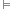In propositional logic, a contradiction is a formula which implies two opposing conclusions. A contradiction is always false, and any contradictory formula implies its own negation.

The symbol ⊥ is used to indicate a contradiction. We may formally indicate that a formula φ is contradictory by φ.

We may easily show that the formula (P ∧ ¬P) is a contradiction by means of a truth table:

P P ∧ ¬P
T F
F F

Contradictions are often used in formal proofs to demonstrate that the true valuation of a specific proposition or formula leads to a contradiction, and that, therefore, it is false and its negation is true. This method is known as reductio ad absurdum (reduction to absurdity), or proof by contradiction.

The negation of a contradiction is, itself, a tautology.